# FSK with the Si5351 Clock Generator (Increasing the Si5351 register update rate)

The Si5351 spec sheets are complicated and confusing, but in the Arduino universe there are many libraries available for the it.  I started out with the Adafruit one, but have modified it to better suit my needs.  Several modifications were required to allow register updates at my 2.4 KHz interrupt rate.  Others were required, or desired, to clean up some other issues.

Speed-ups:

• Increase the nominal Arduino I2C rate from 100 KHz to 400 KHz (the Si5351 supports a 400 KHz I2C rate).
• The Adafruit library uses individual I2C “write single byte” operations, requiring that the I2C bus start, send an address byte, a data byte, and stop per each byte written.  Instead, where possible I use the I2C “burst write” mode which greatly reduces the number of I2C cycles when updating a register bank.
• When only the PLL “B” numerator is changed, we don’t have to update all the PLL divider registers.  This speeds up the update.

Not particularly speed-related, but the Adafruit library performs a PLL reset when the dividers are updated.  This is not necessary, and creates frequency glitches.  I removed the reset operation when updating divisors.  This also does speed things up a bit.

Not speed-related, but the Adafruit library uses floating-point when calculating the fractional divider register values.  No doubt this is because the Si5351 app note shows the use of the floating-point “floor()” function in the calculation, but this is not required.  When the calculations are performed in the proper order, simple integer math is completely accurate.

Here is the gist of the code I use to update the PLL divider numerator.  Note the use of floor() in the original Adafruit comments (sorry about the line-wrapping):

```void setupPLLnumerator(si5351PLL_t pll, uint8_t a, uint32_t b, uint32_t c) {

uint32_t P1; /* PLL config register P1 */
uint32_t P2; /* PLL config register P2 */
uint32_t P3; /* PLL config register P3 */

/* Feedback Multisynth Divider Equation
*
* where: a = mult, b = num and c = denom
*
* P1 register is an 18-bit value using following formula:
*
* 	P1[17:0] = 128 * mult + floor(128*(num/denom)) - 512
*
* P2 register is a 20-bit value using the following formula:
*
* 	P2[19:0] = 128 * num - denom * floor(128*(num/denom))
*
* P3 register is a 20-bit value using the following formula:
*
* 	P3[19:0] = denom
*/

uint32_t f;
f   = (128 * b) / c;

// build the registers to write
P1 = 128 * a + f - 512;
P2 = 128 * b - f * c;
P3 = c;

// since c (denom) hasn't changed, there's no need to write first two bytes// bytes to be written = 6
uint8_t reg_bank[] = {
//(P3 & 0xFF00) >> 8,          // Bits [15:8] of MSNx_P3 in register 26
//P3 & 0xFF,
(P1 & 0x030000L) >> 16,
(P1 & 0xFF00) >> 8,          // Bits [15:8]  of MSNx_P1 in register 29
P1 & 0xFF,                   // Bits [7:0]  of MSNx_P1 in register 30
((P3 & 0x0F0000L) >> 12) | ((P2 & 0x0F0000) >> 16), // Parts of MSNx_P3 and MSNx_P1
(P2 & 0xFF00) >> 8,          // Bits [15:8]  of MSNx_P2 in register 32
P2 & 0xFF                    // Bits [7:0]  of MSNx_P2 in register 33
};

/* Get the appropriate starting point for the PLL registers */
uint8_t baseaddr = (26); // PLLA

return();
}
```

The timer-tick ISR runs at 2.4 KHz (416.66us). With these changes to the Si5351 library code, updating the PLL numerator takes 241us. The long Gaussian filter takes 50us per cycle, and the other operations in the ISR take less than 5us, so that leaves about 120us (per 2.4 KHz cycle) free for other program activities.  This is comfortably adequate, but trying to run the ISR at a faster rate might prove difficult.

Here is an alternate Si5351 programming library.  I was inspired to improve my I2C functions after looking at this one: https://github.com/etherkit/Si5351Arduino

# FSK with the Si5351 Clock Generator (Gaussian FSK)

Now that we know how to set the Si5351 to generate the FSK frequencies, we need to see about filtering (smoothing) the frequency transitions.

HF FM, using SSB:

• Audio FSK signal modulates SSB transmitter, creating upper-sideband signal. This is identical to FM
• Even with no explicit shaping, SSB transmitter audio filtering shapes the FM transient response
• WSPR and APRS have no filtering
• FT8/JS8 specify Gaussian filter, “BT = 2”, -3dB @ 12.5 Hz

With the Si5351 directly generating the transmit frequency there is no smoothing of the frequency shifts; the frequency change is a step function.  While this can still be received and decoded, the frequency steps create significant in-band interference: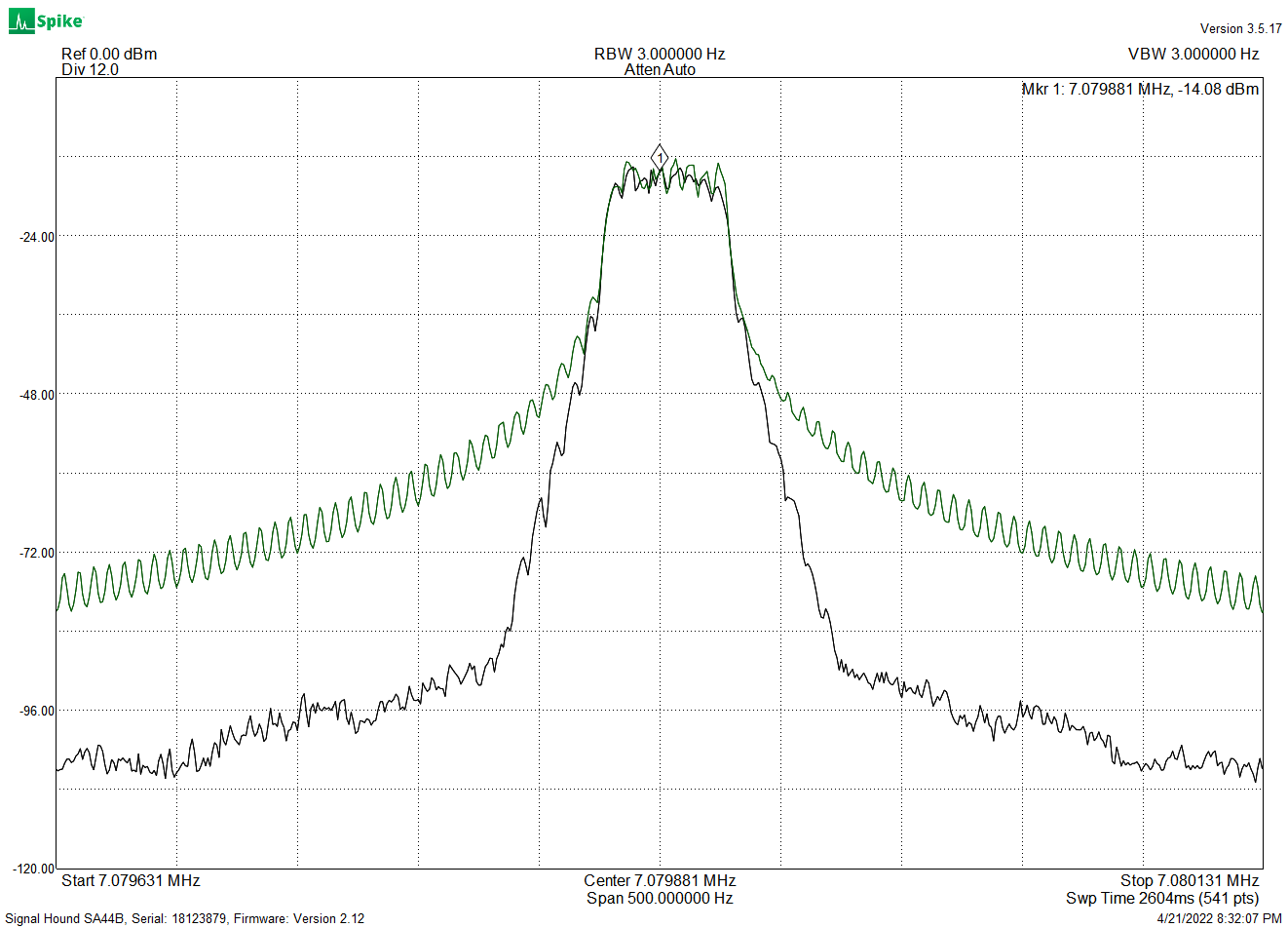FT8 spectrum with and without filtering

In addition, in some cases the receiver uses a filter that is matched to the transmit filter, and having mismatched filters impairs effective signal detection.

The filter specified for FT8 is a Gaussian filter.  This has a specific response, with characteristics that give good spectrum utilization.  These filters have a fairly gentle shape, smoother than a simple RC filter: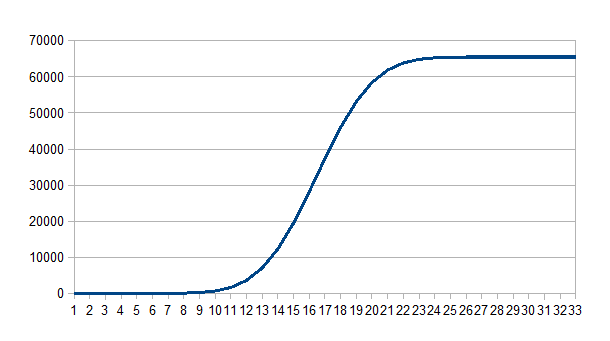Gaussian Filter Step Response

Since we can’t use analog frequency-transition smoothing, we have to do this digitally, using small discrete frequency steps spread out over time.  The Si5351 divisors give us the small frequency steps, and the Drift Buoy software uses a timer-tick interrupt running at a 2.4 KHz frequency to chop the given Baudrate into many small timesteps.

The Gaussian filter runs at this 2.4 KHz rate and using the large frequency steps as an input, generates a smoothed sequence of small frequency steps, which are used to update the  Si5351 dividers.  This filter is implemented in software as a Finite Impulse Response filter (FIR).

The “standard” FIR looks like this: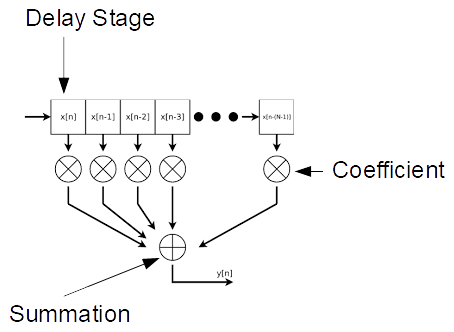The input data is shifted through the delay-register chain, and at each shift the register contents are multiplied by the fixed coefficients and summed, giving the output.  Many different filter responses can be obtained by selection of coefficients.

The Drift Buoy uses a simpler FIR structure, requiring no multiplications (there is one division operation for output scaling):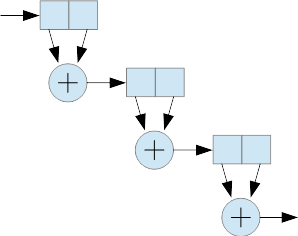Each stage consists of two delay elements (these are 32-bit integer variables) and an addition.  Stages are cascaded as shown, and the resulting filter response is Gaussian.  The FT8 filter is clocked at 2.4 KHz and has 384 stages, which gives a -3dB response of 12.5 Hz.  The filter function takes about 50us per sample.

In the case of FT8, there are 40 available frequency steps from one of the eight tones to the next.  FT8 transmit data generates the eight tone values (0, 1, 2 … 7) . These are mapped to the values (0, 40, 80 … 280), which are sent to the filter.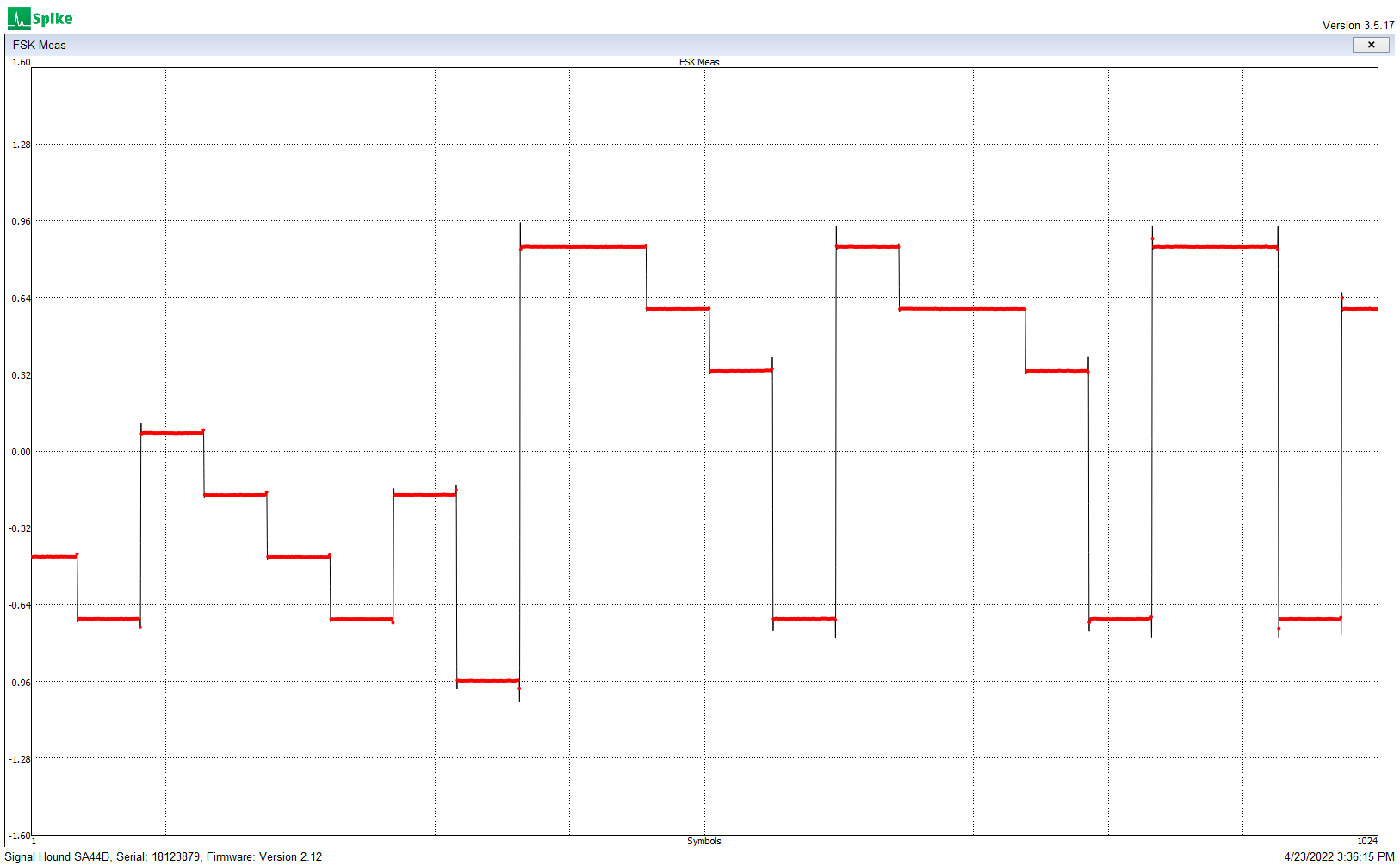Unfiltered frequency steps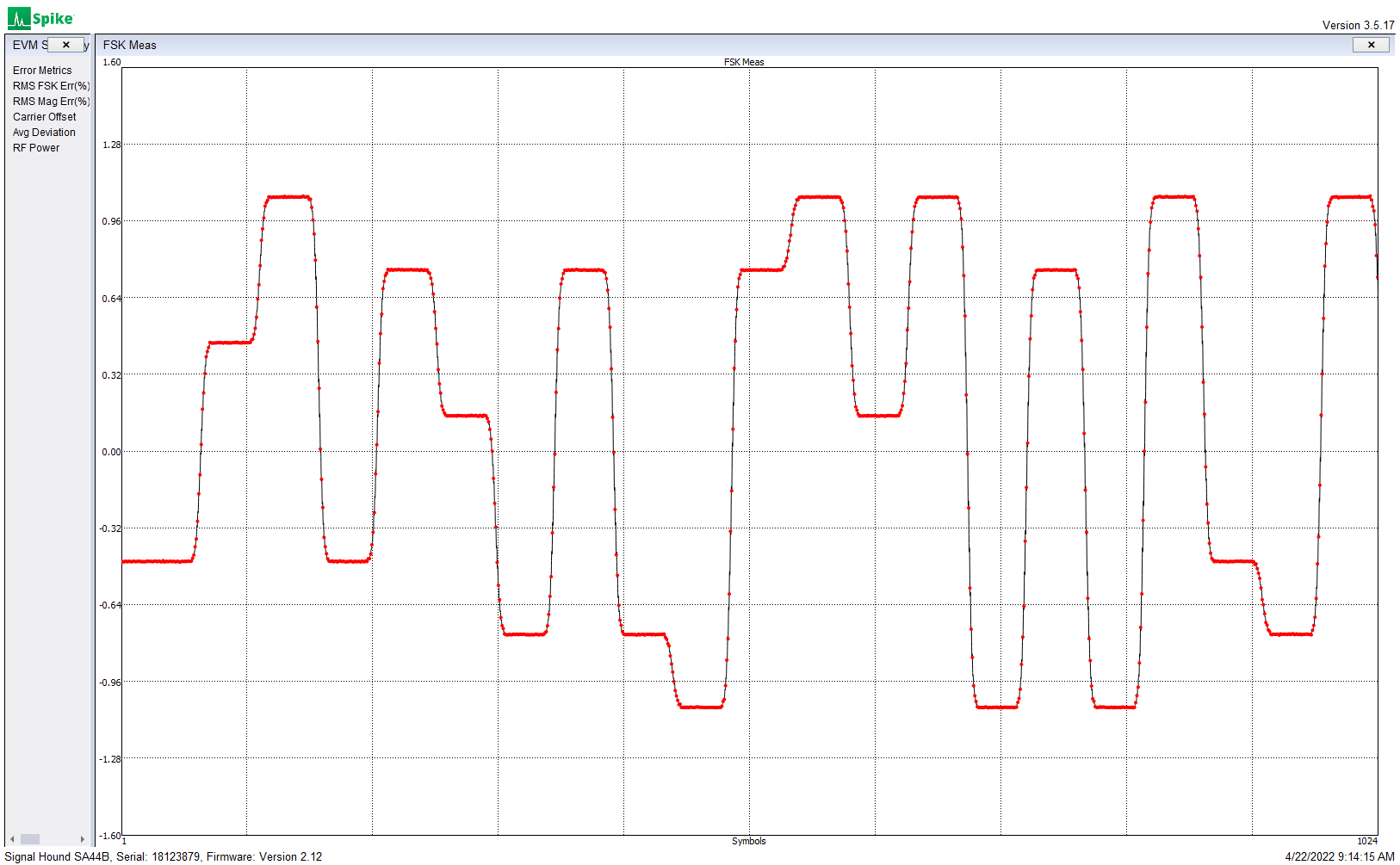Gaussian filtered frequency steps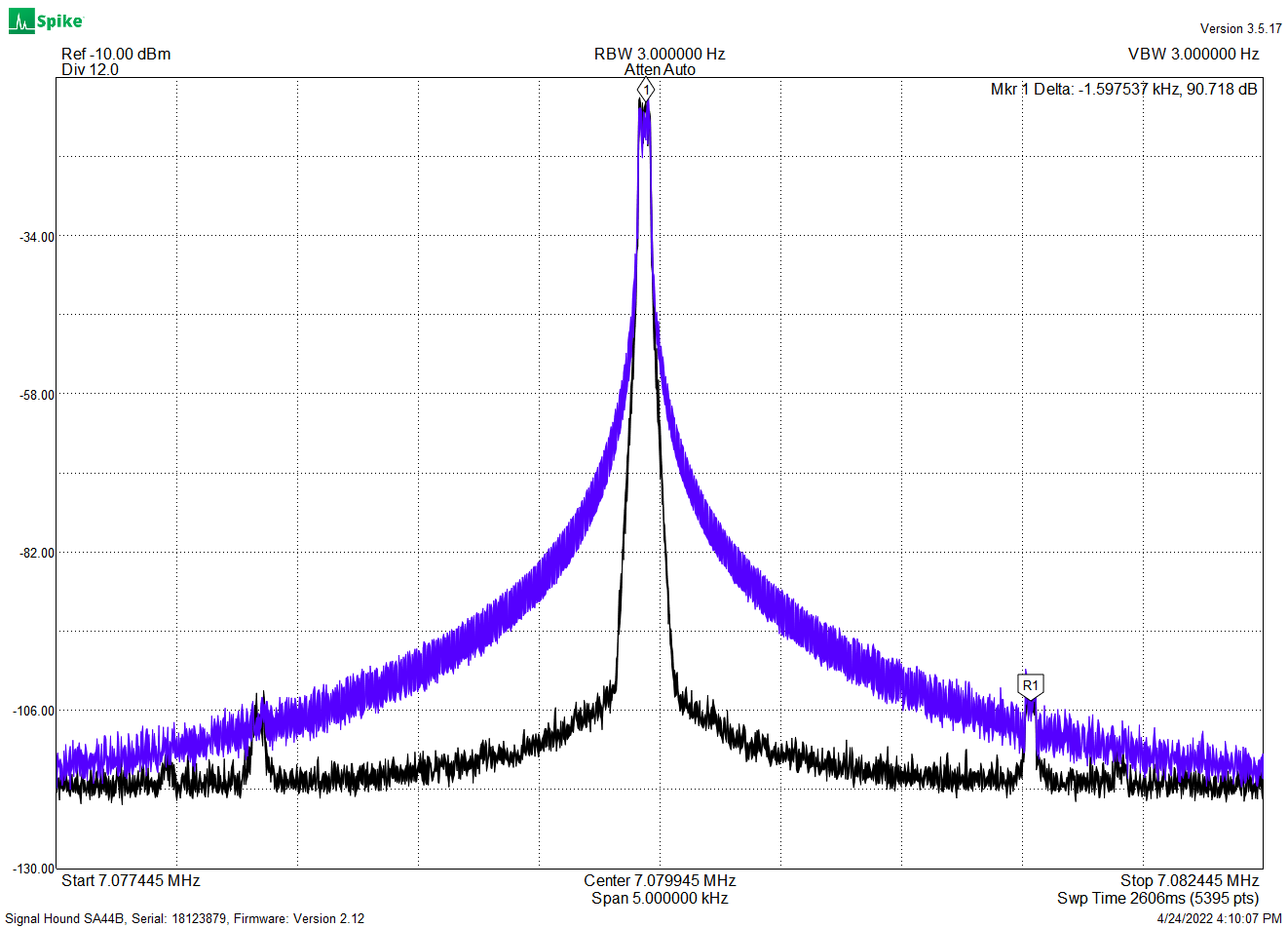Unfiltered (blue) and filtered (black) 8-FSK spectrum,
showing 1,6 KHz sample-rate artifacts

The above plot shows the performance of an earlier version of the FIR, which was running at a 1.6 KHz sample rate.  Increasing this rate to 2.4 KHz pushed the sampling artifact further out and reduced its amplitude.  Updating the Si5351 at the 2.4 KHz rate required speeding up the I2C interface and optimization of the register updates — more on this to follow.

While WSPR and APRS have no filtering requirement, for these modes the Drift Buoy uses a four-stage Gaussian FIR, clocked at 2.4 KHz, to provide filtering similar to the audio bandwidth of a SSB transmitter.

Next:

# FSK with the Si5351 Clock Generator (Fractional Dividers)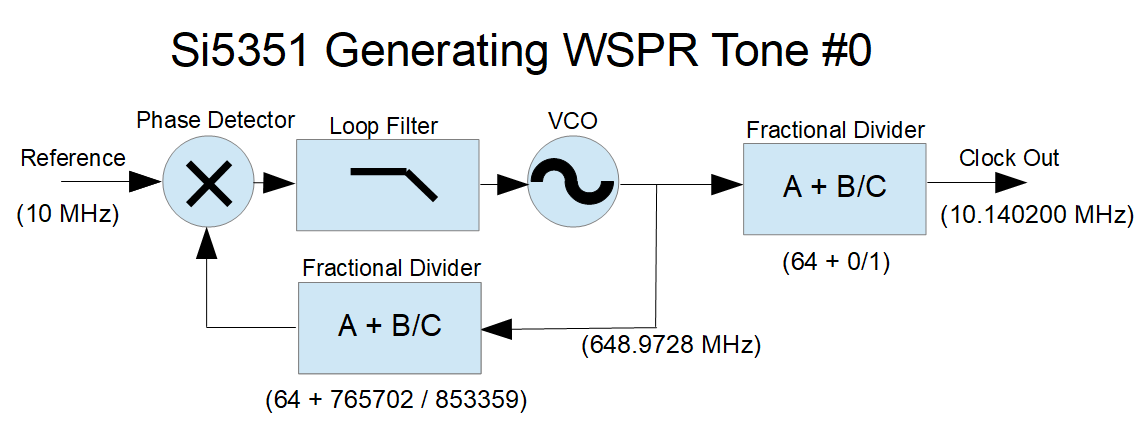This is a simplified illustration of the Si5351, as configured to to generate the WSPR frequencies used in the  Drift Buoy design.

The reference comes from a 10 MHz TCXO, which provides acceptable stability.  The TCXO has an initial frequency accuracy of +/- 2.5ppm, which gives a +/- 25 Hz accuracy at the 10 MHz transmit frequency.  Better initial frequency accuracy and secondary digital temperature compensation can be achieved in software, but this is not necessary for Drift Buoy operation

The output divider is programmed to divide the PLL VCO frequency by 64 (no fractional component), which provides the cleanest spectral output.  The output divisor must be chosen so that the PLL frequency is within the available 600-900 MHz range. The FSK is done using the PLL feedback divider to vary the PLL frequency.

Determining the  PLL divider values is fairly simple.  We start by taking the desired output frequency (in this case 10.1402 MHz) and multiplying it by the output divider value (here, 64).  This gives us a PLL frequency of 648.9728 MHz.  We then take this PLL frequency and find the feedback divisor that will give us our reference frequency (here, a divisor of 64.89728 matches our 10 MHz reference).

So how do we set our “A + B/C” fractional divider to give us a ratio of 64.89728?  The “A” value is easy, that’s just 64.  We could set B = 897,728 and C = 1,000,000 — that would work, and setting C to one million makes the math easy.  But WSPR FSK tone spacing of 1.4648 Hz requires precise frequency control, and with a denominator of 1,000,000, incrementing the numerator by one gives a frequency change of 0.15625 Hz and you can’t get a 1.4648 step with that increment.  You can get close, probably close enough, but with a little math we can select divisor values that work much better.

Here’s a useful (very simple) equation for finding a PLL fractional-divider denominator when you are searching for a particular frequency step:

Fx = reference oscillator frequency in Hz,
OutDiv = output stage divisor.  This can be an integer or a fractional division,
Fdelta= desired output frequency step in Hz.
PLLdenom = PLL fractional divider denominator (the “c” in a + b/c)

Here’s the relationship.  Fdelta= Fx / (OutDiv * PLLdenom).

Rearranging this, we get:  PLLdenom = Fx / (OutDiv * Fdelta).

So for WSPR,  we have:

• Fdelta = 1.4648
• OutDiv = 64
• Fx = 10e6

Which results in PLLdenom = 106669.8525

Since the “C” denominator can only hold integer values, we could round up to 106670, but we can do better.  The “C” value in the Si5351 fractional divider can be any value up to 1,048,575 (which is 2^20 – 1), so we can multiply this PLLdenom by 8, giving a rounded-up “C” of 853,359 (we could also multiply by 9 and still stay within the limits, but for some unknown reason I am using 8).  With this numerator we have a minimum frequency step of 0.18309996 Hz.  Incrementing the “B” numerator by 8 gives steps of 1.46479969 Hz — well within a microHz of the WSPR spec.  We will use these small steps later, when we do Gaussian filtering of the FSK modulation.

So what about the “B” numerator?  With B set to zero, the output frequency will be Fx * A  / (output divider), or in this case 10MHz * 64 / 64, or 10 MHz.  To get the desired 10.1402 MHz we need to set the “B” numerator to  (10,140,200 - 10,000,000) / 0.183099961, which equals 765702 (rounded).  Alternately, we can take the original 64.89728 divisor from our first calculation, and multiply the fractional part by  C/1,000,000 which also equals 765702 (rounded).

There are ways to achieve even finer frequency resolution, by changing both the “B” and “C” values — see the “Farey Sequence” — but the simple method used here requires only one parameter change, allowing for faster configuration of the Si5351 (more on that later.)

This example was for WSPR modulation, but the principles apply to the other FSK modes.

# FSK with the Si5351 Clock Generator (Overview)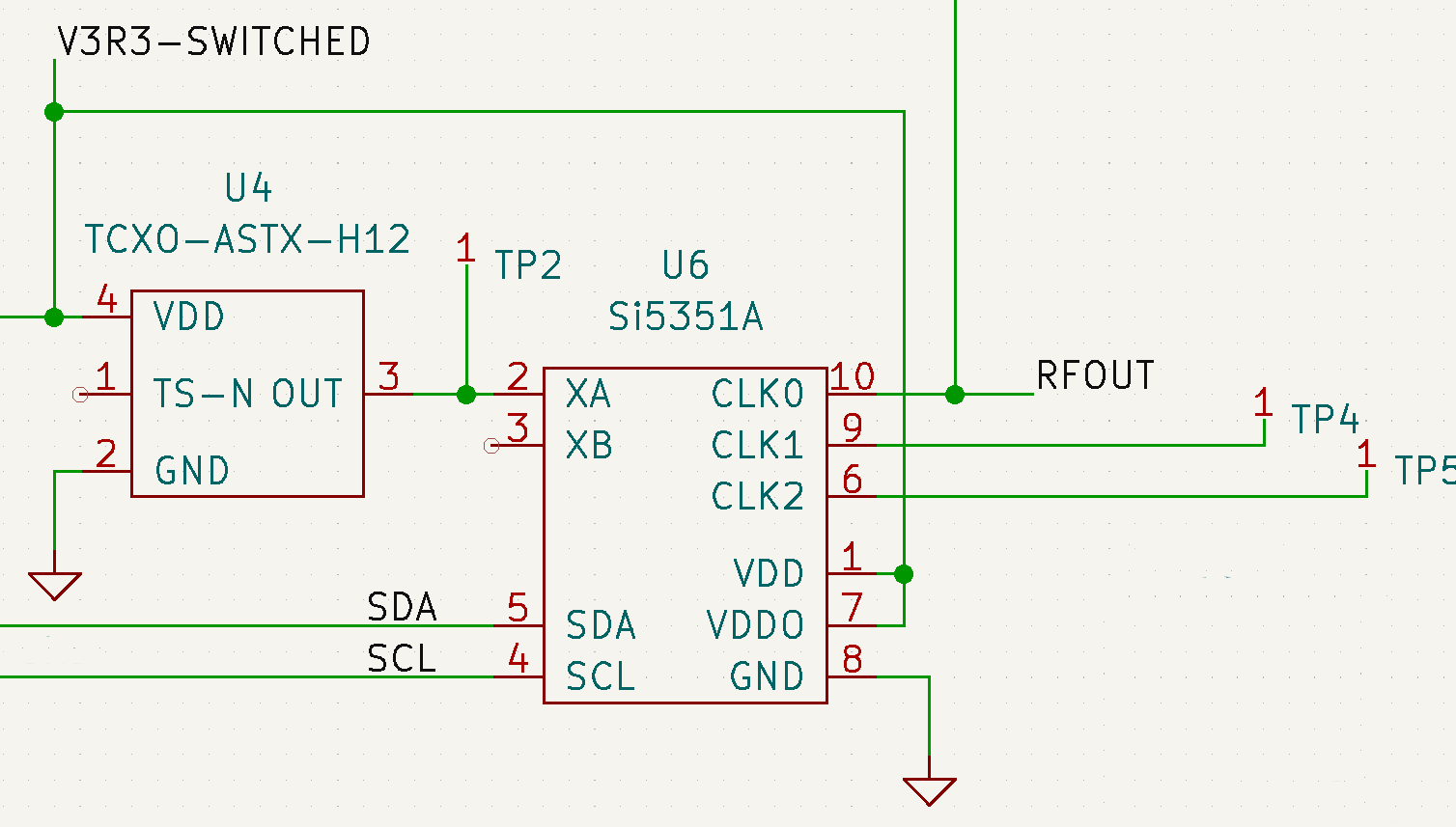The Si55351 Clock Generator chip  is a real workhorse.  Give it a clock reference  (10 – 40 MHz), or a crystal (25 – 27 MHz) and it can generate three different output frequencies, between 2.5 KHz and 200 MHz.  There are two internal PLLs that can run from 600 MHz to 900 MHz, with fractional synthesis feedback dividers that can provide extremely high resolution.  Each of the three output pins is driven by a fractional divider, and a smoothing filter that significantly reduces the frequency transients caused by the digital dividers, providing quite a spectrally pure output for such an inexpensive device (about \$1).  There are more features such as spread-spectrum dithering, and phase offset control (the phase offset can be used to drive quadrature modulator and demodulator architectures.)

With the fractional dividers used in both the PLL feedback and the three output stages, with some restrictions milli-Hertz frequency resolution can be obtained over the full frequency range.   Frequency accuracy is determined by the accuracy of the reference or crystal input. Configuration of the chip is done using a I2C interface.

In the drift-buoy I am using the Si5351 to generate the 30-meter (10.10 – 10.15 MHz) CW and FSK carrier frequencies.  The 3.3V logic level output of this chip feeds a small 1W class-E power amplifier, which drives a short whip antenna.  The FSK modes being used are APRS (2-FSK, 300 Baud, +/- 100 Hz), WSPR (4-FSK, 1.4648 Baud, 1.4648 Hz tone spacing), and FT8/JS8 (8-FSK, 6.25 Baud, 6.25 Hz tone spacing).

Given all the options and flexibility of the chip, there are many ways to generate a specific output frequency.  When generating the FSK frequencies there are many factors to consider.  In the following posts I will cover:

• Selecting and setting the fractional divider values
• Generating Gaussian Frequency Shift Keying
• Increasing the Si5351 register update rate

# Fun With JS8

It’s been a while, but I am returning to the Drift Buoy, and using the JS8 APRS interface for the telemetry.

Things to work out:

• Physical packaging
• Solar / battery / power control
• QRP transmitter power-stage design
• ultra-short antenna matching
• uController and transmitter synthesis/driver
• GPS, environmental sensors
• Program that builds JS8 frames

I’ve made progress here and there on this list, but found myself stumped by the JS8 messaging.  Fortunately the JS8Call program is open-source and the author (Jordan Scherer) is happy to discuss it.  I’ve been able to use the JS8Call program to send APRS EMAIL messages, and using the JS8Call API have captured the “symbol list” that shows what FSK tones are being generated for each frame (messages may take several frames).  I have written a simple “JS8 Explorer” program (borrowing and re-coding much from the JS8Call program) that converts this symbol list to bits.  The Explorer then:

• Strips off the framing fields
• Unscrambles the Forward Error Correction
• Strips off the CRC bits

We now have a 75-bit frame that can be decoded into its various bits and pieces.  There are several types of frame.  I am still figuring out many of the details, but am continuing to hack away at it.

Here’s a screenshot of JS8 Explorer decoding a “Dense Code” frame: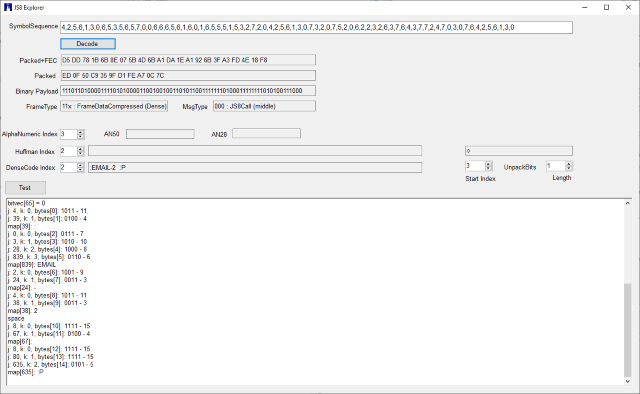Presentation (pdf)

This is a presentation I will be giving at the San Juan County Amateur Radio Society on Friday, April 12.  It describes the JS8 mode and JS8Call program, and the design goals and implementation of an inexpensive JS8 receiver and internet gateway.

# Yet Another WSPR Presentation

Here’s a new presentation I gave to the San Juan County Amateur Radio Society: http://wb6cxc.com/?p=86

This has less detail about Raspberry Pi direct frequency synthesis, but more info on other WSPR projects and some newer technology.  Some of this is going to be designed into a drift-buoy I am contemplating, and I discuss some of the related issues, including ham telemetry.

# WSPR / Raspberry Pi / SDR Presentation

I recently gave a little presentation at the San Juan Amateur Radio Society meeting, about WSPR using the Raspberry Pi transmitter and SDR receivers.  Here are the slides I used: http://wb6cxc.com/?page_id=65

# Weak Signal Reception

I noticed something interesting when I was testing my Raspberry Pi WSPR transmitter:  just how little signal is needed to receive WSPR! I was using a FUNcube Dongle Pro+ receiver to monitor my transmitted signal.  This neat SDR (Software Defined Radio) covers from 150 KHz to 1.9 GHz (with a gap between 240 and 420 MHz).  It has some nice built-in filters and performs well.

The FUNcube was feeding the WSPR WSJT-X program (WSJT-X), and connected to the RPi WSPR transmitter / antenna with a -50dB tap/attenuator:

I made this attenuator a while back when I was testing my 100-Watt ham HF transceiver.  This tap lets me connect the rig to a dummy load (or an antenna) and siphons off a low-level signal that I can connect to my spectrum analyzer or other measuring equipment.  With a 100W through-signal, the signal at the tap is 1mW (that’s -50dB).  With that 100W signal the 7886 Ohm resistor dissipates about 0.6W, so I paralleled four 1/4W resistors (3300 || 3300 || 3300 || 2700 Ohms) to get close to the calculated value.  The thing works well enough at HF frequencies, but I wouldn’t trust the accuracy at VHF.

Anyway, with this tap I could monitor my on-the-air 20-meter WSPR transmissions.  What really surprised me was when I started receiving WSPR signals from the K6SRO WSPR beacon through that 50dB attenuator!   K6SRO transmits at 5W at a distance of over 1,000 kilometers from my receiver.  The received signal-to-noise ratio was -17dB, which is at least 12dB better than needed for WSPR decoding.

I suppose this reminds us that on the HF bands the atmospheric background noise is usually the dominant factor, and when it comes to receiving our antennas are less critical that we might imagine.

# Raspberry Pi WSPR Transmitter – Update

I was fairly astounded to see the difference in spurious signal generation between the two versions of the WsprryPi .  Here’s the output from https://github.com/8cH9azbsFifZ/WsprryPi: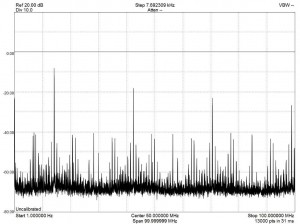And here is the significantly cleaner output from https://github.com/JamesP6000/WsprryPi: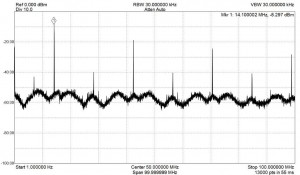What caused the reduction in spurious outputs?  I’ve been studying the programs, and while I still haven’t figured out a lot of the details, one significant difference is the addition of this bit of code:

while (n_pwmclk_transmitted<n_pwmclk_per_sym) {
// Number of PWM clocks for this iteration
long int n_pwmclk=PWM_CLOCKS_PER_ITER_NOMINAL;
// Iterations may produce spurs around the main peak based on the iteration
// frequency. Randomize the iteration period so as to spread this peak
// around.
n_pwmclk+=round((rand()/((double)RAND_MAX+1.0)-.5)*n_pwmclk)*1;
if (n_pwmclk_transmitted+n_pwmclk>n_pwmclk_per_sym) {
n_pwmclk=n_pwmclk_per_sym-n_pwmclk_transmitted;

}

This code snippet is located at line 300 in this file: https://github.com/JamesP6000/WsprryPi/blob/master/wspr.cpp

There may be other relevant changes to the code, but this looks like the big one when it comes to output spur generation.  I will play with it, as well as digging into the rest of the code, and report back.